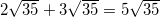## Example Questions

### Example Question #7 : Square Roots And Operations

Find the sum: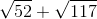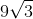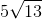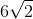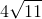Explanation:

Find the Sum: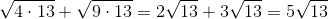### Example Question #11 : Square Roots And Operations

Add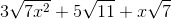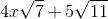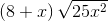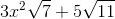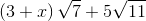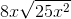Explanation:

The first step when adding square roots is to simplify each term as much as possible. Since the first term has a square within the square root, we can reduce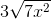to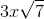. Now each term has only prime numbers within the sqaure roots, so nothing can be further simplified and our new expression is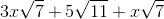.

Only terms that have the same expression within the sqaure root can be combined. In this question, these are the first and third terms. When combining terms, the expression within the square root stays the say, while the terms out front are added. Therefore we get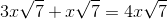. Since the second term of the original equation cannot be combined with any other term, we get the final answer of.

### Example Question #1 : Square Roots And Operations

Simplify: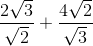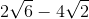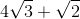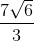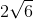Explanation:

Take each fraction separately first:

(2√3)/(√2) = [(2√3)/(√2)] * [(√2)/(√2)] = (2 * √3 * √2)/(√2 * √2) = (2 * √6)/2 = √6

Similarly:

(4√2)/(√3) = [(4√2)/(√3)] * [(√3)/(√3)] = (4√6)/3 = (4/3)√6

√6 + (4/3)√6 = (3/3)√6 + (4/3)√6 = (7/3)√6

### Example Question #4 : How To Add Square Roots

Simplify the following expression: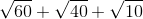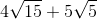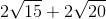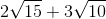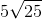Explanation:

Begin by factoring out each of the radicals: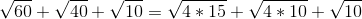For the first two radicals, you can factor out a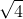or: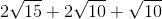The other root values cannot be simply broken down. Now, combine the factors with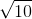: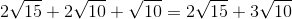### Example Question #11 : Square Roots And Operations

Solve for.

Note,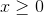: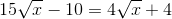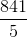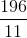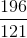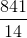Explanation:

Begin by getting yourterms onto the left side of the equation and your numeric values onto the right side of the equation: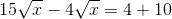Next, you can combine your radicals. You do this merely by subtracting their respective coefficients: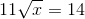Now, square both sides: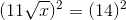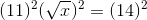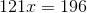Solve by dividing both sides by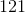: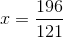### Example Question #12 : Square Roots And Operations

Simplify: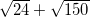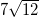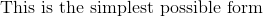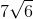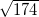Explanation:

Begin simplifying by breaking apart the square roots in question. Thus, you know: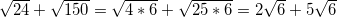Now, with square roots, you can combine factors just as if a given root were a variable. So, just as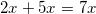, so too does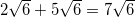.

### Example Question #13 : Square Roots And Operations

Simplify: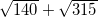.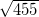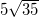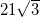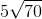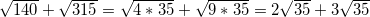Now, with square roots, you can combine factors just as if a given root were a variable. So, just as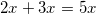, so too does